# P25 Spectrum Sensing with Synthesized and Captured Data

This example shows how to use cyclostationary feature detection to distinguish signals with different modulation schemes, including P25 signals [ 1 ]. It defines four cases of signals: noise only, C4FM, CQPSK, and one arbitrary type. The example applies the detection algorithm to signals with different SNR values, and to a captured real-world P25 signal, and then classifies the signals as one of the four types. Graphical results show that the detection algorithm succeeds in all the cases.

### Project 25 (P25)

Project 25 (P25 or APCO-25) is a suite of standards for digital radio communications for use by federal, state, province and local public safety agencies in North America. When emergencies arise, this protocol suite enables communication among government agencies and mutual aid response teams. In this regard, P25 fills the same role as the European Terrestrial Trunked Radio (TETRA) [ 2 ] protocol, although the two standards are not interoperable with each other. In North America, P25 is widely used in public safety, security, public service, and commercial applications [ 1 ].

Project 25 is deployed in two phases. In Phase 1, P25 uses C4FM, an acronym for compatible 4 level frequency modulation. In its simplest form, it is a special type of 4FSK modulation, which uses four different frequencies to represent symbols. Phase 1 uses this modulation scheme to transmit digital information over a 12.5 kHz channel.

Phase 2 transmits digital information over a 6.25 kHz channel using the compatible quadrature phase shift keying (CQPSK) modulation format. CQPSK modulation is essentially pi/4 differential quadrature phase shift keying (pi/4 DQPSK), where encoding is symmetric, using phase change values of -135 degrees, -45 degrees, +45 degrees and +135 degrees, as shown in the following figure.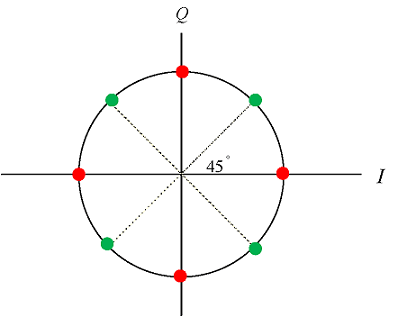In this figure, the next state of the red dots can only be green dots, and vice versa. Although the data rate and bits per symbol are identical, the main difference between the two modulation schemes is that C4FM uses a frequency shift to depict a symbol, which provides a fixed amplitude signal. In contrast, CQPSK, uses a phase shift to depict a symbol, which imparts an amplitude component to the signal.

### Cyclostationary Feature Detection

Modulation recognition and signal classification has been a subject of considerable research for over two decades. Classification schemes can generally be separated into one of two broad categories: likelihood-based (LB) approaches and feature-based (FB) approaches [ 3 ]. Cyclostationary feature detection is an FB technique based on the fact that communications signals are not accurately described as stationary, but rather more appropriately modeled as cyclostationary [ 4 ].

A cyclostationary process is a signal having statistical properties that vary cyclically with time [ 5 ]. These periodicities occur for signals in well defined manners due to processes such as sampling, scanning, modulating, multiplexing, and coding. This resulting periodic nature of signals can be exploited to determine the modulation scheme of the unknown signal [ 4 ].

Cyclostationary feature detection is a robust spectrum sensing technique because modulated information is a cyclostationary process, while noise is not. As a result, cyclic detectors can successfully operate even in low SNR environments.

### Noise-only Case

For the noise-only case, generate a (4*N)-by-1 vector of white Gaussian noise with a power of 1 dBW. 1/(4*N) is the cyclic resolution used to calculate the spectral autocorrelation function (SAF) in commP25ssca.m.

```N = 4096; input = wgn(4*N,1,1); ```

Use the time domain spectral autocorrelation function to analyze the cyclostationary features of the signal x(t). Run the spectral autocorrelation function commP25ssca.m on the input signal. This function estimates the ideal spectral autocorrelation function using the strip spectrum correlation algorithm (SSCA) [ 3 ] temporal smoothing method. It is an FFT based time smoothing algorithm. Refer to [ 6 ] for more information about the implementation of this algorithm.

Run the plot function commP25plot.m. This step illustrates the spectral autocorrelation function, which is a three-dimensional figure. Its x-axis represents the cyclic frequency (alpha) from -1 to 1. Its y-axis represents the spectral frequency (f) from -0.5 to 0.5, and its z-axis (Sx) represents the corresponding magnitude of the spectral autocorrelation function for each (alpha , f) pair. Cyclic resolution dalpha = 1/T, where T is the observation time of the data. Spectral resolution df = 1/Tw, where Tw is the window time to calculate the complex demodulate [ 7 ]. Since T > Tw, dalpha < df. Note that when alpha does not equal zero, the SAF values are approximately zero.

```% 64 represents the window time Tw, 4*N represents the observation time T [Sx,alphao,fo] = commP25ssca(input,1,1/64,1/(4*N)); fig1 = figure('Position',figposition([5 40 40 40])); commP25plot(Sx,alphao,fo); ```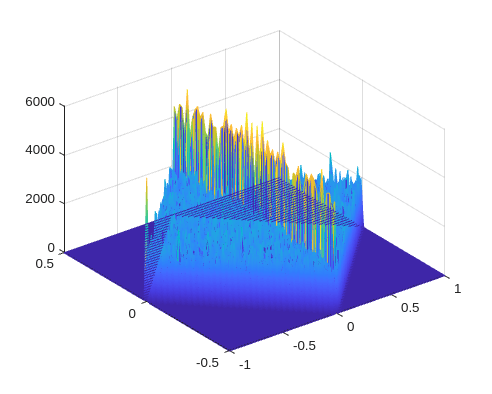commP25decision_noise.m determines if the input signal contains only noise. commP25decision_c4fm.m determines if the input signal is a C4FM signal. And commP25decision_cqpsk.m determines if the input signal is a CQPSK signal. These decisions are based upon the location of the peaks in the SAF. In this example, the code correctly concludes that there is no P25 signal present.

```[c,d] = size(Sx); [Ades,Index] = sort(Sx(:),'descend'); % sort Sx by its element and store in Ades [Ridx,Cidx] = ind2sub(size(Sx),Index); % corresponding row index and column index leng = length(Ades); noise_decision = commP25decision_noise(Ades,Ridx,Cidx,leng,c,d); if noise_decision == 0 c4fm_decision = commP25decision_c4fm(Ades,Ridx,leng,c); if c4fm_decision == 0 commP25decision_cqpsk(Ades,Ridx,Cidx,leng,c,d); end end ```
```There is no P25 signal. ```

### C4FM Case with Synthesized Data

According to [ 8 ], the following modulation structure generates a C4FM output signal.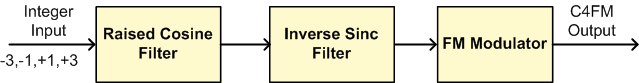A normal raised cosine filter, which satisfies the Nyquist pulse shaping criterion, minimizes intersymbol interference. The parameters of the raised cosine filter are chosen per the filter's specifications in [ 8 ]. Specifically, this raised cosine filter has an upsampling factor of 4, and a roll-off factor of 0.2. The C4FM standard also calls for an inverse sinc filter after the raised cosine filter, to compensate for the sinc response of a P25 receiver integrate and dump filter. The FM modulator has a deviation of 600 Hz.

To observe the effects of noise on the design decisions, run the detection at SNR values of -3 dB, 3 dB and infinity dB.

```% The length of input bits is N. The length of the output bits must also be % N x = randi([0,3],N,1); sym = 2*x-3; % integer input % Raised Cosine Filter sampsPerSym = 4; % Upsampling factor % Design raised cosine filter with given order in symbols. Apply gain to % the unit energy filter to obtain max amplitude of 1. rctFilt = comm.RaisedCosineTransmitFilter(... 'Shape', 'Normal', ... 'RolloffFactor', 0.2, ... 'OutputSamplesPerSymbol', sampsPerSym, ... 'FilterSpanInSymbols', 60, ... 'Gain', 1.9493); c4fm_init = rctFilt(sym); shape2 = 'Inverse-sinc Lowpass'; d2 = fdesign.interpolator(2, shape2); intrpltr = design(d2, 'SystemObject', true); c4fm_init = intrpltr(c4fm_init); % Baseband Frequency Modulator Fs = 4800; freqdev = 600; int_x = cumsum(c4fm_init)/Fs; c4fm_output = exp(1i*2*pi*freqdev*int_x); y = c4fm_output(1:N); % Ideal case, SNR = infinity y1 = awgn(y,3); % SNR = 3 dB y2 = awgn(y,-3); % SNR = -3 dB ```

The corresponding spectral autocorrelation functions are calculated and plotted. Note that the SAF peaks become more indistinct as the SNR decreases.

```[Sx0,alphao0,fo0] = commP25ssca(y,1,1/64,1/(4*N)); [Sx1,alphao1,fo1] = commP25ssca(y1,1,1/64,1/(4*N)); [Sx2,alphao2,fo2] = commP25ssca(y2,1,1/64,1/(4*N)); fig2 = figure('Position',figposition([5 40 80 40])); subplot(131); commP25plot(Sx0,alphao0,fo0); title('Ideal case'); subplot(132); commP25plot(Sx1,alphao1,fo1); title('SNR = 3 dB'); subplot(133); commP25plot(Sx2,alphao2,fo2); title('SNR = -3 dB'); ```This section follows the same procedures as in the previous one and obtains the classification results for each SNR value. The function commP25decision.m performs spectrum sensing classification for all possible input signal types.

```commP25decision(Sx0); % Ideal case ```
```There is signal present. Checking for presence of C4FM. This is C4FM. ```
```commP25decision(Sx1); % SNR = 3 dB ```
```There is signal present. Checking for presence of C4FM. This is C4FM. ```
```commP25decision(Sx2); % SNR = -3 dB ```
```There is signal present. Checking for presence of C4FM. This is C4FM. ```

### CQPSK Case with Synthesized Data

According to [ 8 ], the following modulation structure generates a CQPSK output signal.The CQPSK modulator consists of In Phase and Quadrature (I and Q) parts. The input bits are processed by the lookup table [ 8 ] to yield a 5-level I/Q signal. Because the specification of the lookup table is equivalent to pi/4 DQPSK, the example uses the DQPSK modulator System object™ to implement this lookup table. The I/Q signals are then filtered with the raised cosine filter described in the previous case.

```% The size of input bits is 2*N, the size of output is 4*N x = randi([0,1],2*N,1); % Create a DQPSK modulator System object(TM) with bits as inputs, phase % rotation of pi/4 and Gray-coded constellation dqpskMod = comm.DQPSKModulator(pi/4,'BitInput',true); % Modulate and filter modout = dqpskMod(x); release(rctFilt); cqpsk_output = rctFilt(modout); y = cqpsk_output; % Ideal case, SNR = infinity y1 = awgn(y,3); % SNR = 3 dB y2 = awgn(y,-3); % SNR = -3 dB ```

Calculate and plot the corresponding spectral autocorrelation functions.

```[Sx0,alphao0,fo0] = commP25ssca(y,1,1/64,1/(4*N)); [Sx1,alphao1,fo1] = commP25ssca(y1,1,1/64,1/(4*N)); [Sx2,alphao2,fo2] = commP25ssca(y2,1,1/64,1/(4*N)); fig3 = figure('Position',figposition([5 40 80 40])); subplot(131); commP25plot(Sx0,alphao0,fo0); title('Ideal case'); subplot(132); commP25plot(Sx1,alphao1,fo1); title('SNR = 3 dB'); subplot(133); commP25plot(Sx2,alphao2,fo2); title('SNR = -3 dB'); ```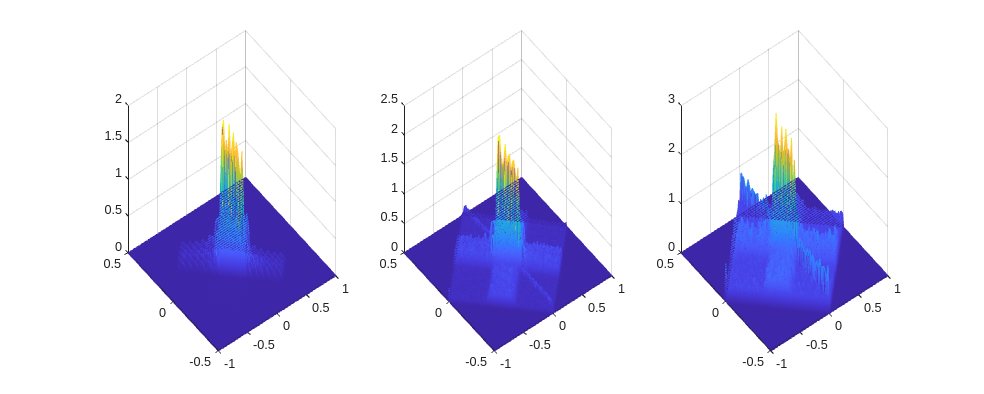The code outputs below show the results of CQPSK detection for three different SNR values.

```commP25decision(Sx0); % Ideal case ```
```There is signal present. Checking for presence of C4FM. This is NOT C4FM. Checking for presence of CQPSK. This is CQPSK. ```
```commP25decision(Sx1); % SNR = 3 dB ```
```There is signal present. Checking for presence of C4FM. This is NOT C4FM. Checking for presence of CQPSK. This is CQPSK. ```
```commP25decision(Sx2); % SNR = -3 dB ```
```There is signal present. Checking for presence of C4FM. This is NOT C4FM. Checking for presence of CQPSK. This is CQPSK. ```

### Non-P25 Signal Case with Synthesized Data

This case defines one arbitrary signal type, processes it with the P25 cyclostationary detector, and determines if it is a P25 signal.

Design an FIR equiripple lowpass filter, and apply it to a random input. Do not add any noise to the signal in this case. Try additional signal types, and let the cyclostationary feature detector classify them.

```bcoeffs = firpm(200,[0 0.2 0.22 1],[1 1 0 0]); % Set N to achieve 40 dB rejection input = randn(N,1); y = filter(bcoeffs,1,input); ```

Then we calculate and plot the spectral autocorrelation function. Note that the different modulation characteristics of each signal yield significantly different SAFs.

```[Sx,alphao,fo] = commP25ssca(y,1,1/64,1/(4*N)); fig4 = figure('Position',figposition([5 40 40 40])); commP25plot(Sx,alphao,fo); ```Follow the same procedures and obtain the classification result.

```commP25decision(Sx); ```
```There is signal present. Checking for presence of C4FM. This is NOT C4FM. Checking for presence of CQPSK. This is NOT CQPSK either, so it is not a P25 signal. ```

### C4FM Case with Captured Data

This case applies the detection algorithm to a captured real-world C4FM signal. The signal was transmitted by a P25 radio at 446 MHz, received by a USRP™ radio, and then saved by MATLAB® in capturedc4fm.mat. Follow the same procedures and obtain the classification result.

```load capturedc4fm.mat; y = y(1:4*N); agc = comm.AGC; y = 0.1*agc(y); [Sx,alphao,fo] = commP25ssca(y,1,1/64,1/(4*N)); fig5 = figure('Position',figposition([5 40 40 40])); commP25plot(Sx,alphao,fo); commP25decision(Sx); ```
```There is signal present. Checking for presence of C4FM. This is C4FM. ```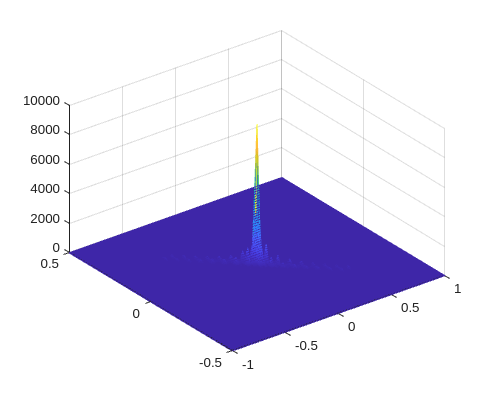### Conclusion

This example shows how to use cyclostationary feature detection to distinguish signals of different modulation schemes. The algorithm classifies the signals based on the location of the peaks in spectral autocorrelation function. Cyclostationary feature detection has advantages over some detectors, like the energy detector, due to its resilience to noise.

### Appendix

This example uses the following scripts and helper functions:

### Selected Bibliography

1. P25 Technology Interest Group: http://www.project25.org/

2. E. C. Like, "Non-Cooperative Modulation Recognition Via Exploitation of Cyclic Statistics". MS Thesis. 2007

3. E. C. Like, V. D. Chakravarthy, P. Ratazzi, and Z. Wu, "Signal Classification in Fading Channels Using Cyclic Spectral Analysis", EURASIP Journal on Wireless Communications and Networking, Volume 2009, 2009.

4. W. A. Gardner, A. Napolitano and L. Paura, "Cyclostationarity: Half a century of research", Signal Processing, Vol. 86, No. 4, pp. 639-697, 2006.

5. E. L. Da Costa, "Detection and Identification of Cyclostationary Signals". MS Thesis. 1996.

6. Antonio F. Lima, Jr., "Analysis of Low Probability of Intercept (LPI) Radio Signals using Cyclostationary Processing". MS Thesis. 2002.

7. TIA Standard Project 25: <https://www.tiaonline.org/what-we-do/standards/>